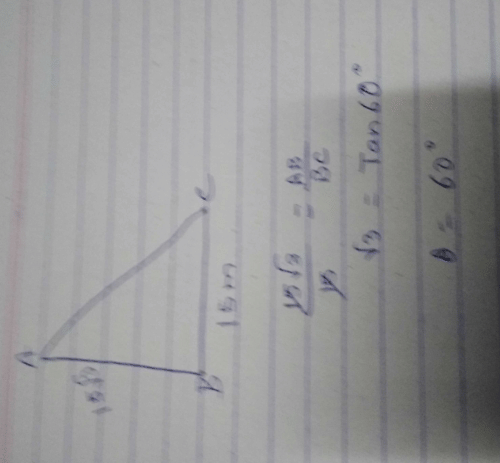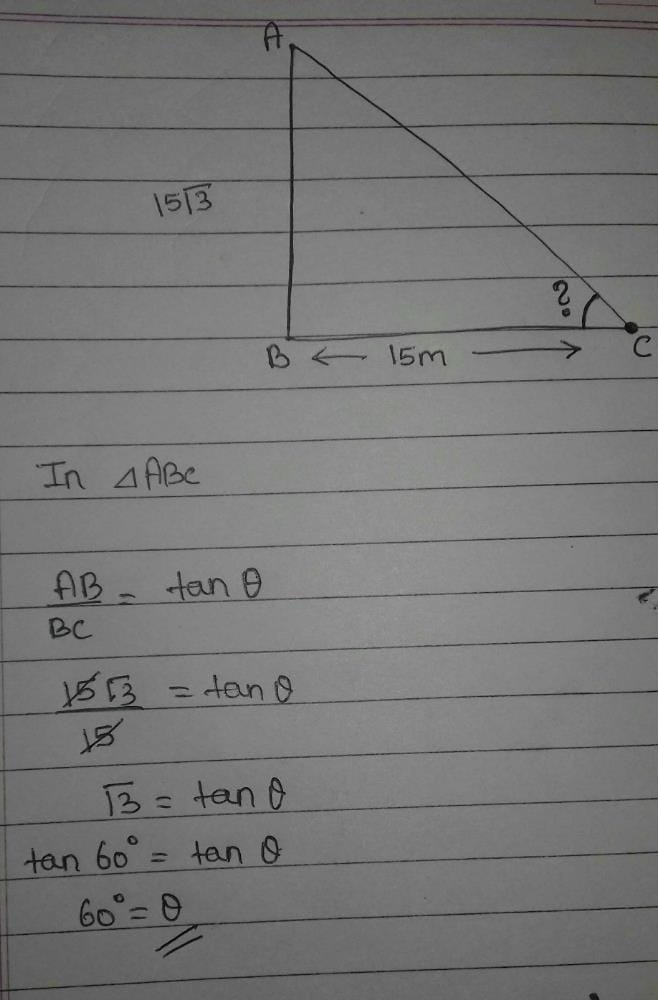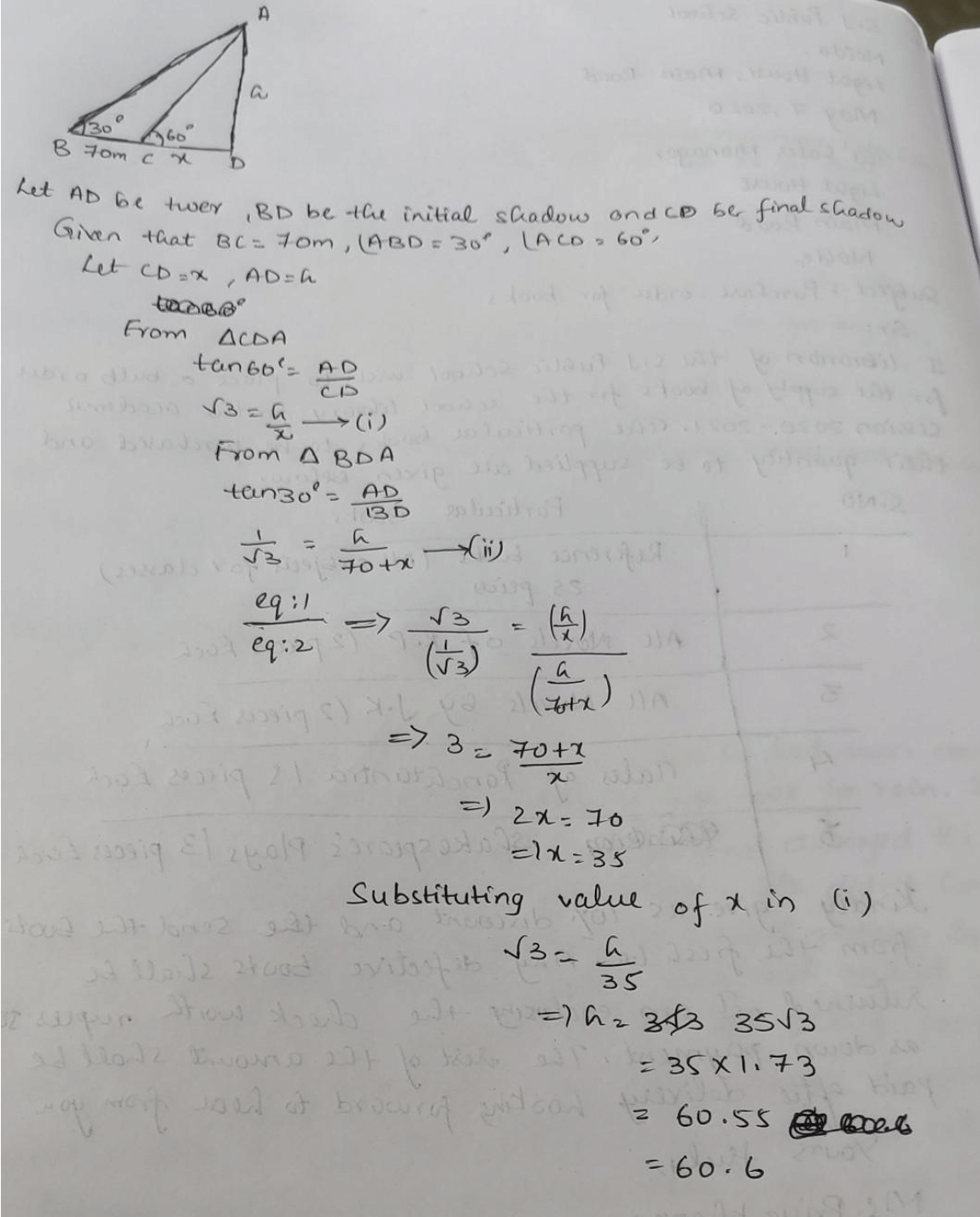A tower stands vertically on the ground from ...

### Related TestA tower stands vertically on the ground from a point on the ground which is 15 m away from the foot of tower. If the height of tower is 15√3 meters find the angle of elevation.​
• a)
120°
• b)
60°
• c)
30°
• d)
90°Sushant Jha1 Crore+ students have signed up on EduRev. Have you?Himaja Ammu
A tower stands vertically on the ground from a point on the ground which is 15 m away from the foot of tower. If the height of tower is 15√3 meters find the angle of elevation.​a)120°b)60°c)30°d)90°Correct answer is 'B'. Can you explain this answer?View courses related to this question Explore Class 10 courses
 Explore Class 10 coursesView courses related to this questionEshikaMoviez Klaser
A tower stands vertically on the ground from a point on the ground which is 15 m away from the foot of tower. If the height of tower is 15√3 meters find the angle of elevation.​a)120°b)60°c)30°d)90°Correct answer is 'B'. Can you explain this answer?
Let required angle @.
tan@=15×root3/15
=root3
but tan60=root3

therefore tan@=tan60
or,@=60EduRev Class 10Question Description
A tower stands vertically on the ground from a point on the ground which is 15 m away from the foot of tower. If the height of tower is 15√3 meters find the angle of elevation.​a)120°b)60°c)30°d)90°Correct answer is 'B'. Can you explain this answer? for Class 10 2023 is part of Class 10 preparation. The Question and answers have been prepared according to the Class 10 exam syllabus. Information about A tower stands vertically on the ground from a point on the ground which is 15 m away from the foot of tower. If the height of tower is 15√3 meters find the angle of elevation.​a)120°b)60°c)30°d)90°Correct answer is 'B'. Can you explain this answer? covers all topics & solutions for Class 10 2023 Exam. Find important definitions, questions, meanings, examples, exercises and tests below for A tower stands vertically on the ground from a point on the ground which is 15 m away from the foot of tower. If the height of tower is 15√3 meters find the angle of elevation.​a)120°b)60°c)30°d)90°Correct answer is 'B'. Can you explain this answer?.
Solutions for A tower stands vertically on the ground from a point on the ground which is 15 m away from the foot of tower. If the height of tower is 15√3 meters find the angle of elevation.​a)120°b)60°c)30°d)90°Correct answer is 'B'. Can you explain this answer? in English & in Hindi are available as part of our courses for Class 10. Download more important topics, notes, lectures and mock test series for Class 10 Exam by signing up for free.
Here you can find the meaning of A tower stands vertically on the ground from a point on the ground which is 15 m away from the foot of tower. If the height of tower is 15√3 meters find the angle of elevation.​a)120°b)60°c)30°d)90°Correct answer is 'B'. Can you explain this answer? defined & explained in the simplest way possible. Besides giving the explanation of A tower stands vertically on the ground from a point on the ground which is 15 m away from the foot of tower. If the height of tower is 15√3 meters find the angle of elevation.​a)120°b)60°c)30°d)90°Correct answer is 'B'. Can you explain this answer?, a detailed solution for A tower stands vertically on the ground from a point on the ground which is 15 m away from the foot of tower. If the height of tower is 15√3 meters find the angle of elevation.​a)120°b)60°c)30°d)90°Correct answer is 'B'. Can you explain this answer? has been provided alongside types of A tower stands vertically on the ground from a point on the ground which is 15 m away from the foot of tower. If the height of tower is 15√3 meters find the angle of elevation.​a)120°b)60°c)30°d)90°Correct answer is 'B'. Can you explain this answer? theory, EduRev gives you an ample number of questions to practice A tower stands vertically on the ground from a point on the ground which is 15 m away from the foot of tower. If the height of tower is 15√3 meters find the angle of elevation.​a)120°b)60°c)30°d)90°Correct answer is 'B'. Can you explain this answer? tests, examples and also practice Class 10 tests.

• ### A TV tower stands vertically on a bank of canal . from a point on the other... more(Scan QR code)